# Program to check Disarium Number In Java

In this tutorial you will learn about the Program to check Disarium Number In Java and its application with practical example.

In this tutorial, we will learn to create a Java Program to check Disarium Numbers in Java using Java programming.

## Prerequisites

Before starting with this tutorial we assume that you are best aware of the following Java programming topics:

• Java Operators.
• Basic Input and Output function in Java.
• Class and Object in Java.
• Basic Java programming.
• If-else statements in Java.
• For loop in Java.
• Inbuilt functions.

## What is In  Disarium Numbers?

A number is called Disarium number if sum of its power at the positions from left to right is equal to the number.

Example:
11 + 32 + 53 = 1 + 9 + 125 = 135

## Program to check Disarium Number In Java

In this program we will find given number is a Disurium number or not in using java programming. We would first declared and initialized the required variables. Next, we would prompt user to input the a number.Later we  find number is Sisarium number or not let’s have a look at the code.

## Output

Disarium Number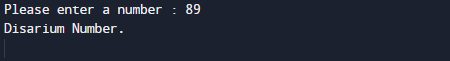Not a Disarium Number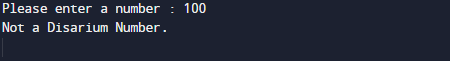In the above program, we have first declared and initialized a set variables required in the program.

• number= it will hold entered number
• temp= it will hold temporary origianal number
• sum= it will hold sum of values
• l= it will hold length of a number

After declaring variables in the next statement user will be prompted to enter a value and  which will be assigned to variable ‘number’.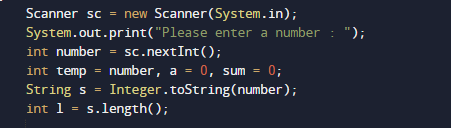And after that we calculate the value Sum of its digits powered with their respective position is equal to the original number or not using Math.pow(a,l). inbuilt function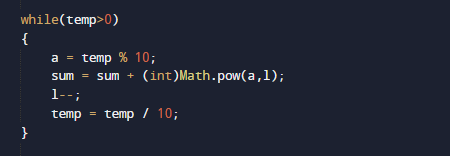So the given number called Disarium if “sum of its digits powered with their respective position are equal” with the number.

Number is Disarium if condition True.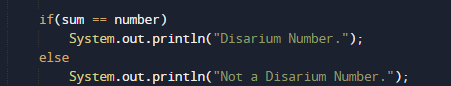If not it is not a Disarium Number.

In this tutorial we have learn about the Program to check Disarium Number In Java and its application with practical example. I hope you will like this tutorial.